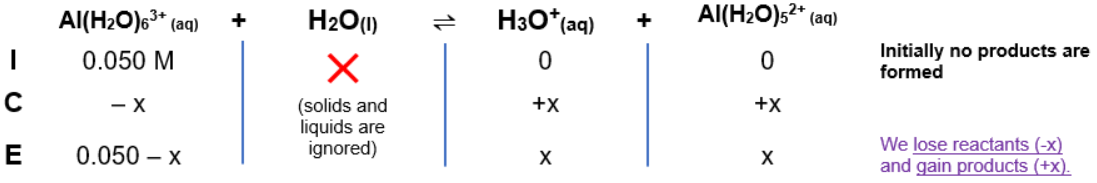# Problem: Calculate the pH of a 0.050-M Al(NO 3)3 solution. The Ka value for Al(H2O)63+ is 1.4 X 10-5.

###### FREE Expert Solution

$\overline{){\mathbf{pH}}{\mathbf{=}}{\mathbf{-}}{\mathbf{log}}{\mathbf{\left[}}{{\mathbf{H}}}_{{\mathbf{3}}}{{\mathbf{O}}}^{{\mathbf{+}}}{\mathbf{\right]}}}$

Species in solution: Al(H2O)63+, NO3-, H2O

• Al(H2O)63+Stronger acid than H2O

Dominant equilibriumAl(H2O)63+(aq) + H2O(l)  H3O+(aq) +Al(H2O)52+(aq)

ICE table:Ka expression:

$\overline{){{\mathbf{K}}}_{{\mathbf{a}}}{\mathbf{=}}\frac{\mathbf{products}}{\mathbf{reactants}}{\mathbf{=}}\frac{\mathbf{\left[}{\mathbf{H}}_{\mathbf{3}}{\mathbf{O}}^{\mathbf{+}}\mathbf{\right]}\mathbf{\left[}\mathbf{Al}{{\mathbf{\left(}\mathbf{H}}_{}}^{}}{}}$2O)52+][Al(H2O)63+]

Solving for x = [H3O+]

94% (457 ratings)###### Problem Details

Calculate the pH of a 0.050-M Al(NO 3)3 solution. The Ka value for Al(H2O)63+ is 1.4 X 10-5.

Frequently Asked Questions

What scientific concept do you need to know in order to solve this problem?

Our tutors have indicated that to solve this problem you will need to apply the Weak Acids concept. If you need more Weak Acids practice, you can also practice Weak Acids practice problems.

What professor is this problem relevant for?

Based on our data, we think this problem is relevant for Professor Cole & Geng & Lovander's class at IOWA.

What textbook is this problem found in?

Our data indicates that this problem or a close variation was asked in Chemistry: An Atoms First Approach - Zumdahl Atoms 1st 2nd Edition. You can also practice Chemistry: An Atoms First Approach - Zumdahl Atoms 1st 2nd Edition practice problems.